Updating search results...

# 83 Results

View
Selected filters:
• Texas Instruments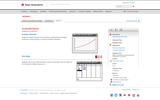Rating
0.0 stars
Overview:

Students compare periodic and continuous compounding and apply continuous compounding to a variety of problem situations.

Subject:
Mathematics
Material Type:
Activity/Lab
Provider:
Texas Instruments
03/05/2018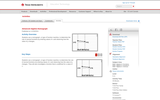Rating
0.0 stars
Overview:

This activity is similar to the idea of a function machine. There are two levels of the manipulative (called a nomograph). The first is comprised of two vertical number lines, input on the left and output on the right. The second has three number lines to accommodate displaying the composition of two functions. At the first level, students try to find the rule of a hidden function by entering domain values and observing how they are transformed to new (range) values. The transformation is illustrated dynamically by an arrow that connects a domain entry to its range value. At the second level, students investigate composite functions. Inverse functions are treated as special cases of composition.

Subject:
Mathematics
Material Type:
Activity/Lab
Provider:
Texas Instruments
03/05/2018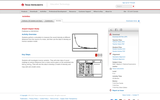Rating
0.0 stars
Overview:

Students perform a simulation to measure the sound intensity at different distances from the source of a noise, and then use the data to develop an inverse variation model.

Subject:
Mathematics
Material Type:
Activity/Lab
Provider:
Texas Instruments
03/07/2018Rating
0.0 stars
Overview:

Texas Instruments calculator activity relating similarity and dilations.

Subject:
Mathematics
Material Type:
Activity/Lab
Provider:
Texas Instruments
03/05/2018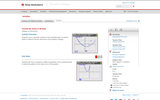Rating
0.0 stars
Overview:

Texas Instruments Activity using TI-83 or 84 series calculator showing domain and range of transformations.

Subject:
Mathematics
Material Type:
Activity/Lab
Provider:
Texas Instruments
03/07/2018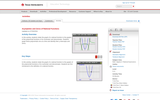Rating
0.0 stars
Overview:

Students relate the graph of a rational function to the graphs of the polynomial functions of its numerator and denominator. Students graph these polynomials one at a time and identify their y-intercepts and zeros.

Subject:
Mathematics
Material Type:
Activity/Lab
Provider:
Texas Instruments
03/07/2018Rating
0.0 stars
Overview:

Texas Instrument Activity. In this activity, students will use TI-83/TI-84 to construct triangle and explore properties of the centroid.

Subject:
Mathematics
Material Type:
Activity/Lab
Provider:
Texas Instruments
03/05/2018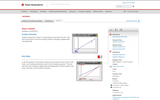Rating
0.0 stars
Overview:

Texas Instruments activity to solve systems of equations.

Subject:
Mathematics
Material Type:
Activity/Lab
Provider:
Texas Instruments
03/05/2018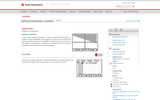Rating
0.0 stars
Overview:

Students will graph one linear inequality and then graph a system of linear inequalities as they apply to an area of land. Students will practice graphing systems of inequalities and determining if a points lies in the solution. In the extension, students are challenged to write a system of inequalities that describe the area of Mississippi.

Subject:
Mathematics
Material Type:
Activity/Lab
Provider:
Texas Instruments
03/07/2018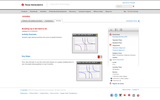Rating
0.0 stars
Overview:

Students split rational functions into sums of partial fractions.

Subject:
Mathematics
Material Type:
Activity/Lab
Provider:
Texas Instruments
03/07/2018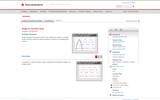Rating
0.0 stars
Overview:

Texas Instruments activity where Students graph a quadratic function that models the shape of a bridge trestle and then solve the related quadratic equation by completing the square.

Subject:
Mathematics
Material Type:
Activity/Lab
Provider:
Texas Instruments
03/07/2018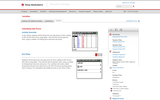Educational Use
Rating
0.0 stars
Overview:

In this activity, students will find discounts and sale prices for items selling at 20% off and 40% off by using tables. They will then find the general rules, using a variable, for finding those discounts and prices.

Subject:
Mathematics
Material Type:
Activity/Lab
Provider:
Texas Instruments
Author:
Texas Instruments, Inc.
04/23/2019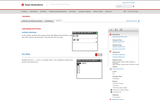Educational Use
Rating
0.0 stars
Overview:

In this activity, students will compare ratios with different denominators, and then with common denominators. They will also find unit rates.

Subject:
Mathematics
Material Type:
Activity/Lab
Provider:
Texas Instruments
Author:
Texas Instruments, Inc.
04/23/2019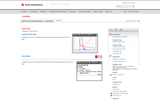Rating
0.0 stars
Overview:

Students will run two experiments that simulate pouring out coins from a bag.

Subject:
Mathematics
Material Type:
Activity/Lab
Provider:
Texas Instruments
03/07/2018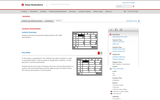Educational Use
Rating
0.0 stars
Overview:

In this activity, students will use the CelSheet App that multiplies the fraction by “1” to help determine the common denominator. Then they will use what they have learned to add and subtract fractions without the same denominators. As an extension, they will use the LCM command to quickly obtain the best denominator to complete the operations.

Subject:
Mathematics
Material Type:
Activity/Lab
Provider:
Texas Instruments
Author:
Texas Instruments, Inc.
04/23/2019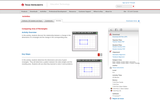Educational Use
Rating
0.0 stars
Overview:

In this activity, students discover the relationship between a change in the dimensions of a rectangle and the change in the corresponding area.

Subject:
Mathematics
Material Type:
Activity/Lab
Provider:
Texas Instruments
Author:
Texas Instruments, Inc.
04/23/2019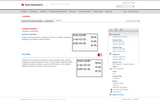Rating
0.0 stars
Overview:

Texas Instruments activity dealing with complex numbers.

Subject:
Mathematics
Material Type:
Activity/Lab
Provider:
Texas Instruments
03/05/2018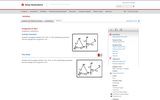Rating
0.0 stars
Overview:

Texas Instruments activity that has students determine if triangles are congruent based on angles and sides.

Subject:
Mathematics
Material Type:
Activity/Lab
Provider:
Texas Instruments
03/07/2018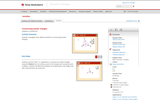Rating
0.0 stars
Overview:

Texas Instruments activity exploring triangle similarity and transformations.

Subject:
Mathematics
Material Type:
Activity/Lab
Provider:
Texas Instruments
03/05/2018Rating
0.0 stars
Overview:

Module includes tasks to conduct on graphing calculator regarding continuity, types of discontinuities and piecewise functions.

Subject:
Mathematics
Material Type:
Activity/Lab
Provider:
Texas Instruments
03/07/2018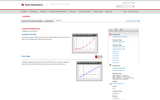Rating
0.0 stars
Overview:

Students are given data, asked to find the finite differences, and then use this to find a polynomial that models the data. Requires TI 83/84 Technology.

Subject:
Mathematics
Material Type:
Activity/Lab
Provider:
Texas Instruments
03/05/2018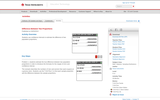Rating
0.0 stars
Overview:

Students use confidence intervals to estimate the difference of two population proportions.

Subject:
Mathematics
Material Type:
Activity/Lab
Provider:
Texas Instruments
03/07/2018Rating
0.0 stars
Overview:

Texas Instruments activity showing dilations.

Subject:
Mathematics
Material Type:
Activity/Lab
Provider:
Texas Instruments
03/07/2018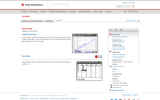Rating
0.0 stars
Overview:

Texas Instruments lesson comparing the cost of three different scenarios for a dinner party and the significance of slope and y intercept in each situation.

Subject:
Mathematics
Material Type:
Activity/Lab
Provider:
Texas Instruments
03/05/2018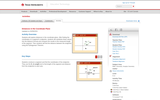Rating
0.0 stars
Overview:

Texas Instruments activity exploring distances in the coordinate plane in order to classify shapes.

Subject:
Mathematics
Material Type:
Activity/Lab
Provider:
Texas Instruments
03/07/2018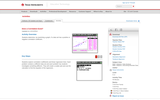Rating
0.0 stars
Overview:

Texas Instruments activity that uses linear regression specifically to calculate a correlation coefficient.

Subject:
Mathematics
Material Type:
Activity/Lab
Provider:
Texas Instruments
03/07/2018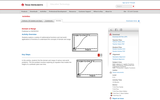Rating
0.0 stars
Overview:

Students explore a variety of mathematical functions and real world applications of functions to understand the concepts of domain and range.

Subject:
Mathematics
Material Type:
Activity/Lab
Provider:
Texas Instruments
03/05/2018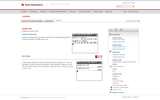Rating
0.0 stars
Overview:

Texas Instruments activity with exponential growth where a tree doubles in growth every year.

Subject:
Mathematics
Material Type:
Activity/Lab
Provider:
Texas Instruments
03/05/2018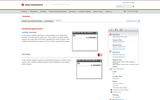Educational Use
Rating
0.0 stars
Overview:

In this activity, students develop an understanding of the relationship between a number and its square root. They will first recognize perfect squares and order them from least to greatest with other numbers. Then students will estimate the square root of a number using perfect squares. Requires TI technology.

Subject:
Mathematics
Material Type:
Activity/Lab
Provider:
Texas Instruments
Author:
Texas Instruments, Inc.
04/23/2019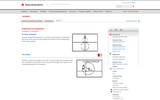Rating
0.0 stars
Overview:

Students will discover the equation of circles of the form (x â€“ h)^2+ (y â€“ k)^2= r^2, where (h, k) is the center of the circle and r is the radius of the circle.

Subject:
Mathematics
Material Type:
Activity/Lab
Provider:
Texas Instruments
03/07/2018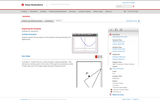Rating
0.0 stars
Overview:

Students explore the key features of the parabola, both geometrically and algebraically.

Subject:
Mathematics
Material Type:
Activity/Lab
Provider:
Texas Instruments
03/05/2018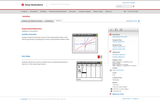Rating
0.0 stars
Overview:

Students will determine that the inverse of the exponential function is the natural log function by plotting the inverse of exponential solution points.

Subject:
Mathematics
Material Type:
Activity/Lab
Provider:
Texas Instruments
03/07/2018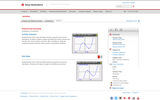Rating
0.0 stars
Overview:

Students learn how to find and label extrema using first and second derivatives, be able to inspect a graph and determine which extrema the function has, and be able to use trace, fMin, and fMax to verify the computed answers and find critical values for parametric functions.

Subject:
Mathematics
Material Type:
Activity/Lab
Provider:
Texas Instruments
03/05/2018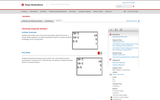Educational Use
Rating
0.0 stars
Overview:

In this activity, students will work with composite numbers to find their prime factorizations in exponent form. They will create factor trees and use division as a means to finding prime factors. An extension involving finding a common denominator for two fractions is given at the end of the activity.

Subject:
Mathematics
Material Type:
Activity/Lab
Provider:
Texas Instruments
Author:
Texas Instruments, Inc.
04/23/2019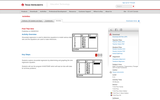Rating
0.0 stars
Overview:

Sinusoidal regression is used to determine equations to model various data sets and the equations are used to make inferences.

Subject:
Mathematics
Material Type:
Activity/Lab
Provider:
Texas Instruments
03/05/2018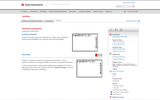Rating
0.0 stars
Overview:

Students simulate a geometric distribution of rolling a die to determine experimental probabilities and calculate theoretical probabilities.

Subject:
Mathematics
Material Type:
Activity/Lab
Provider:
Texas Instruments
03/05/2018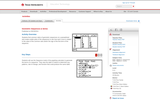Rating
0.0 stars
Overview:

Students find common ratios of geometric sequences on a spreadsheet and create scatter plots of the sequences to see how each curve is related to the value of the common ratio and/or the sign of the first term of the sequence.

Subject:
Mathematics
Material Type:
Activity/Lab
Provider:
Texas Instruments
03/07/2018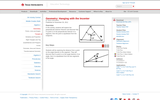Rating
0.0 stars
Overview:

Students will explore the perpendicular bisector theorem and discover that if a point is on the perpendicular bisector of a segment, then the point is equidistant from the endpoints.

Subject:
Mathematics
Material Type:
Activity/Lab
Provider:
Texas Instruments
03/05/2018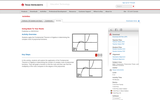Rating
0.0 stars
Overview:

In this activity, students apply the Fundamental Theorem of Algebra in determining the complex roots of polynomial functions. The theorem is applied both algebraically and graphically.

Subject:
Mathematics
Material Type:
Activity/Lab
Provider:
Texas Instruments
03/05/2018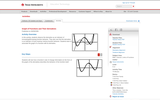Rating
0.0 stars
Overview:

Students observe the derivative as an indicator of increasing/decreasing function behavior. They also see that the derivative is an indicator of local maxima/ minima function behavior. Students learn to associate the graph of a function with its derivative. REQUIRES TI 83/84 TECHNOLOGY

Subject:
Mathematics
Material Type:
Activity/Lab
Provider:
Texas Instruments